SBOS487B June   2009  – March 2020

PRODUCTION DATA.

1. Features
2. Applications
3. Description
1.     Device Images
4. Revision History
5. Pin Configuration and Functions
6. Specifications
7. Detailed Description
1. 7.1 Overview
2. 7.2 Functional Block Diagram
3. 7.3 Feature Description
1. 7.3.1 Functional Blocks
2. 7.3.2 Error Indicators
4. 7.4 Device Functional Modes
1. 7.4.1 GPIO Operation Mode
5. 7.5 Programming
1. 7.5.1 SPI and Register Description
2. 7.5.2 Command Structure and Register Overview
1. 7.5.2.1 Command Byte
2. 7.5.2.2 Extended CS
3. 7.5.3 GPIO Configuration
4. 7.5.4 Buffer Timing
6. 7.6 Register Map
8. Application and Implementation
1. 8.1 Application Information
9. Power Supply Recommendations
10. 10Device and Documentation Support
11. 11Mechanical, Packaging, and Orderable Information

• PW|24

### 6.4 Typical Characteristics

At TA = +25°C, VSP = +15V, VSN = –15V, VSON = 0V, VSOP = 5V, DVDD = +3V, DGND = 0V, RL = 2.5kΩ to VSOP/2 = VOCM, G = 1V/V, using internal clock, BUF inactive, VCM = 0V, and differential input and output, unless otherwise noted.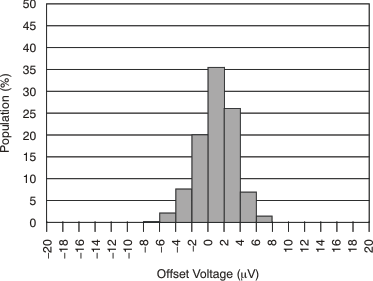Figure 2. Offset Voltage Production Distribution (G = 128)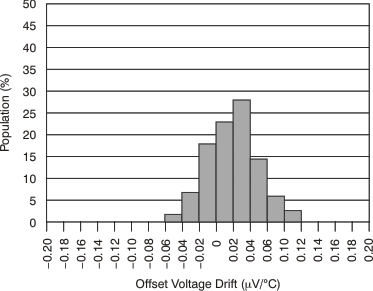Figure 4. Offset Voltage Drift Distribution (G = 128)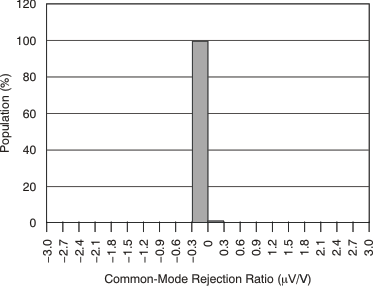Figure 6. Common-Mode Rejection Distribution (G = 128)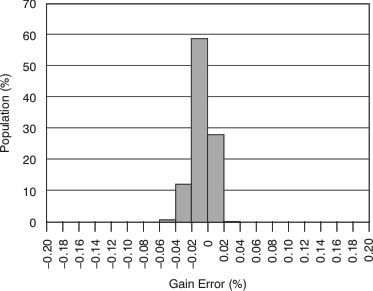Figure 8. Gain-Error Distribution (G = 128)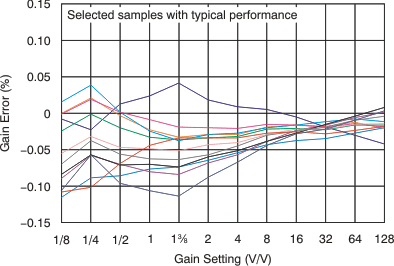Figure 10. Gain Error vs Gain SettingFigure 12. Maximum Gain-Error Deviation Between
Sequential Gain Settings (Mean With ±3 σ)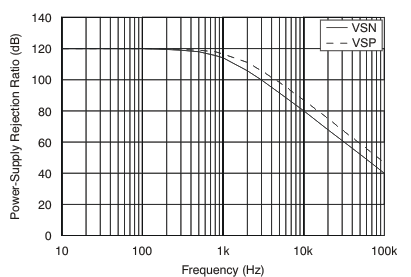Figure 14. Power-Supply Rejection vs FrequencyFigure 16. Input-Referred Noise Spectrum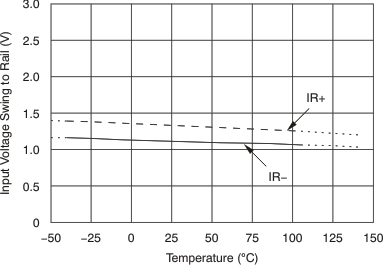Figure 18. Input Voltage Range Limits vs Temperature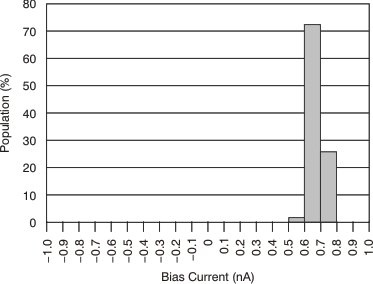Figure 20. Input Bias Current Distribution (G = 128)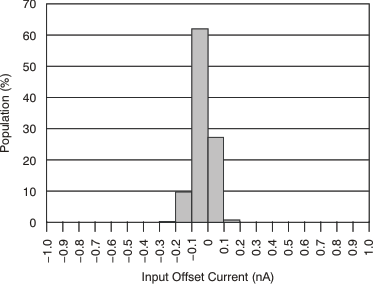Figure 22. Input Offset Current Distribution (G = 1, G = 128)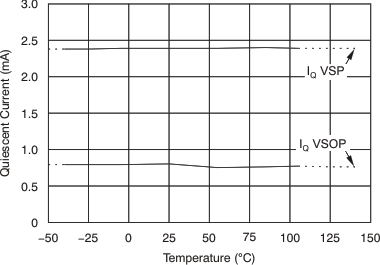Figure 24. Quiescent Current From Supplies (VSP and VSOP) vs Temperature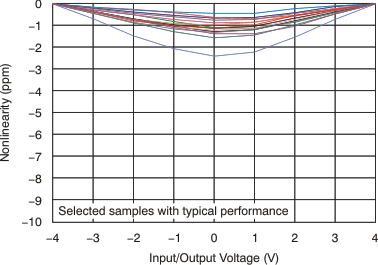Figure 26. Gain Nonlinearity With End-Point Calibration
(G = 1)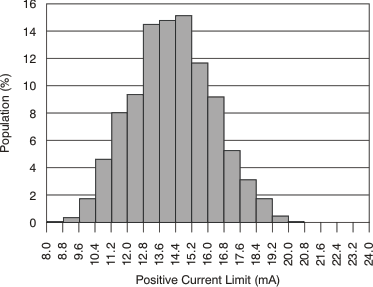Figure 28. Positive Output Current Limit Distribution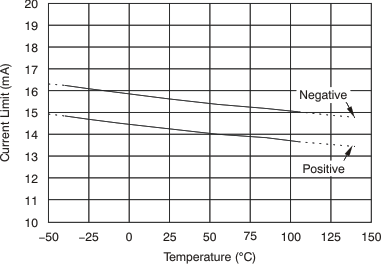Figure 30. Output Current Limit vs Temperature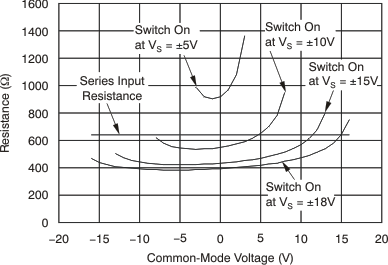Figure 32. Switch-On Resistance
and Series Input Resistance
vs Common-Mode Voltage at Various Supply Voltages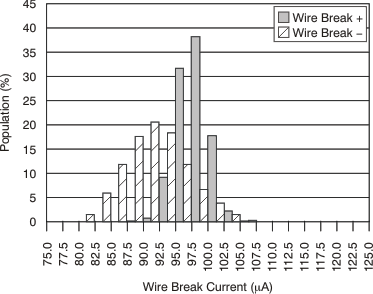Figure 34. Wire Break Current Distribution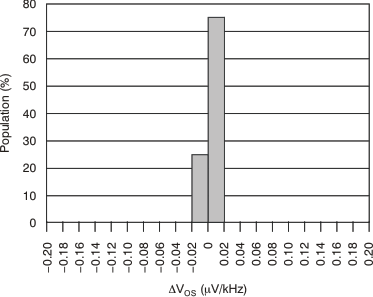Figure 36. Influence of External Clock Frequency to
VOS Performance (G = 128)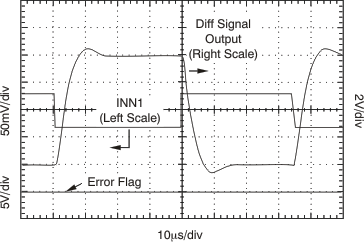Figure 38. Step Response (G = 128)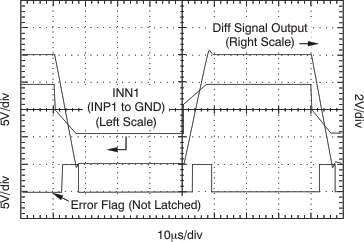Figure 40. Step Response (G = 1)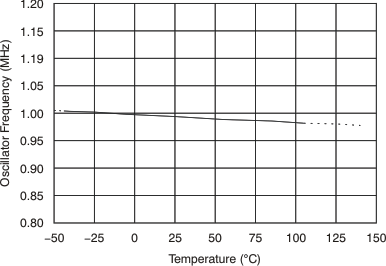Figure 42. Oscillator Frequency vs
TemperatureFigure 3. Offset Voltage Production Distribution (G = 1)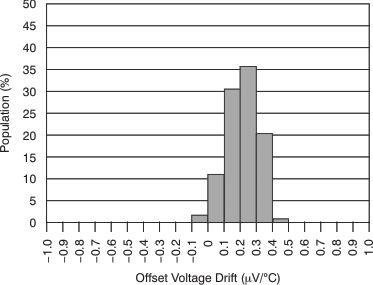Figure 5. Offset Voltage Drift Distribution (G = 1)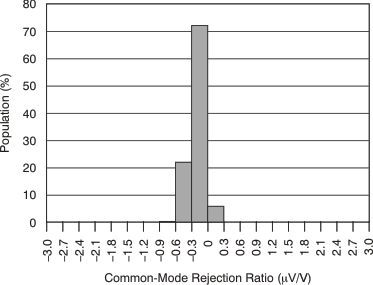Figure 7. Common-Mode Rejection Distribution (G = 1)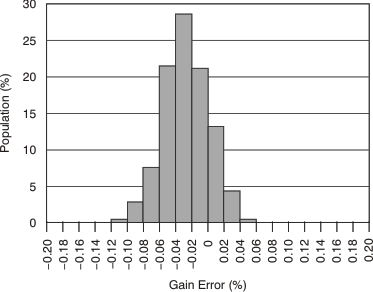Figure 9. Gain-Error Distribution (G = 1)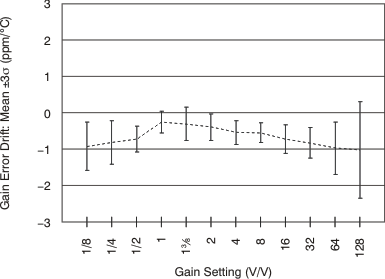Figure 11. Gain-Error Drift Distribution
vs Gain Setting (Mean With ±3 σ)Figure 13. Gain-Error Distribution vs
Gain Setting (Mean With ±3σ)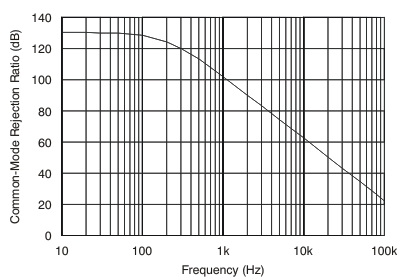Figure 15. Common-Mode Rejection vs Frequency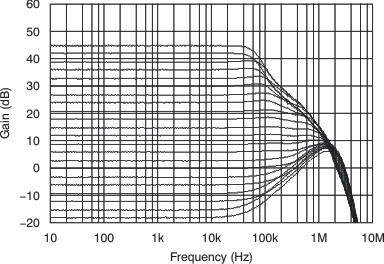Figure 17. Small-Signal Gain vs Frequency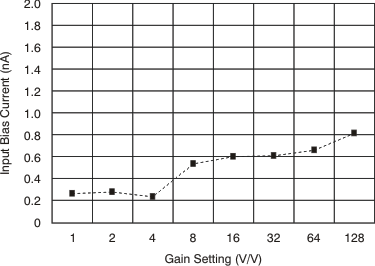Figure 19. Bias Current vs Gain Setting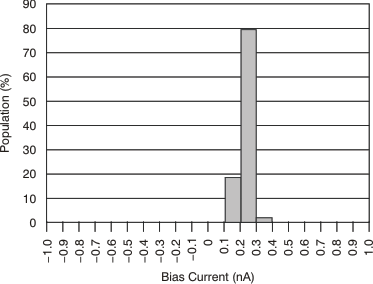Figure 21. Input Bias Current Distribution (G = 1)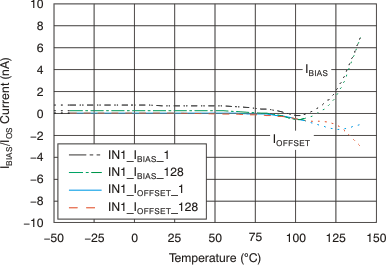Figure 23. Input Bias Current and Input Offset Current
vs Temperature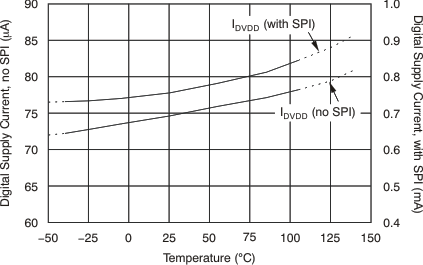Figure 25. Digital Supply Current
With and Without SPI Communication
vs Temperature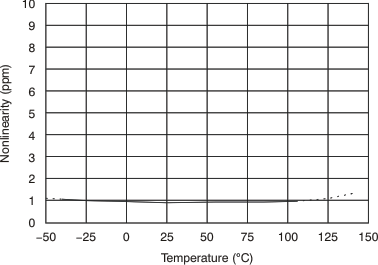Figure 27. Gain Nonlinearity vs TemperatureFigure 29. Negative Output Current Limit Distribution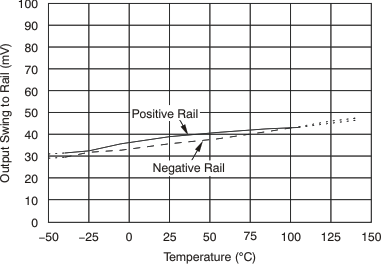Figure 31. Output Swing To Rail vs Temperature
(VSOP – VSON = 5 V)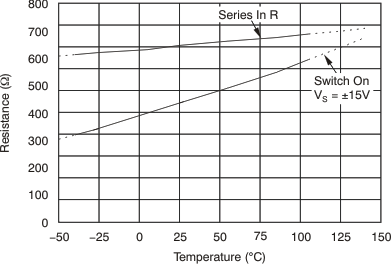Figure 33. Switch-On Resistance
and Series Input Resistance
vs Temperature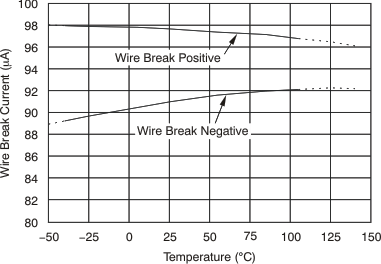Figure 35. Wire Break Current Magnitude vs Temperature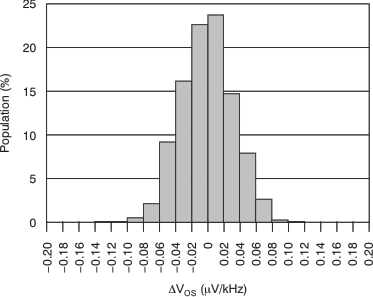Figure 37. Influence of External Clock Frequency to
VOS Performance (G = 1)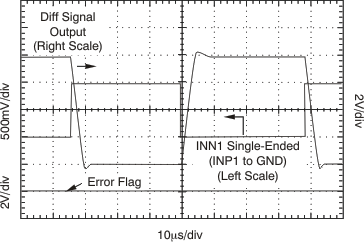Figure 39. Step Response (G = 8)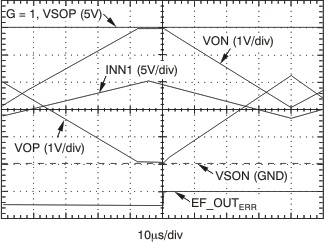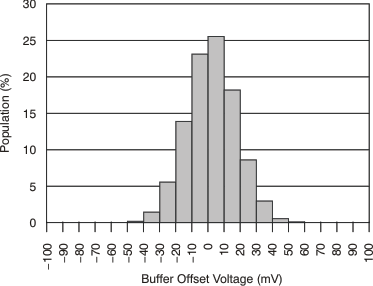Figure 43. Input Current Buffer Offset Voltage Distribution